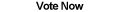Home Articles Components Code Snippets Links# C# Sort 2D Points

## Introduction

How can we sort a list of 2D points in C#? There is no one way to sort a list of points by default, it all depends on which value we are looking at: X or Y.

Sorting respective to the X values will sort a list of points from left to right (or vice versa). Likewise sorting the Y values sorts the points top to bottom.

This is useful when applying more advanced processing algorithms to a list of points. An example is image distortion algorithms, where a list of sorted point corners is crucial.

## Sorting the List of Points

To sort the list of 2D points, we are going to create a C# class that inherits the interface IComparer. The main meat of the function will be the Compare C# function which , depending on whether to sort left to right or top to bottom, will compare the X or Y values of the points.

The actual sorting algorithm is handled by the .NET Framework, all we need to do in the Compare function is establish what makes one point greater than, less than, or equal to a second point.

Here is the header of the C# function:

```int IComparer.Compare(object a, object b)
```

Note that the parameter types are object, this is so the .NET Framework can easily call the function later. Accordingly the objects need to be Pointsbefore we do anything. Simply use (Point)a and (Point)b.

Notice how the function Compare returns an int, the int has to be one of three values:
0 - The two objects are equal
1 - The first object is greater than the second object
-1 - The first object is less than the second object

Then calling the actual sorting is quite simple:

```Array.Sort(pointArray, new PointSort(PointSort.Mode.X));
```

Simple C# code right? Since the actual sorting is done by the .NET Framework, the process has already been optimized to run at top speeds.

The detail I did not include is that the PointSort C# class needs to know by which value (X or Y) to sort by, that's what PointSort.Mode is for.

To see how the whole thing comes together, how to sort a list of 2D Points in C#, download the C# sorting project files.

Back to C# Article List

Other C# Articles